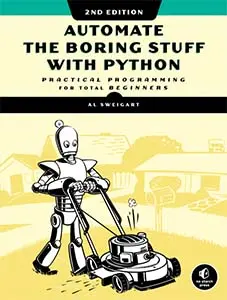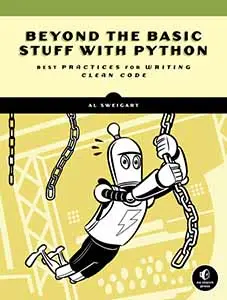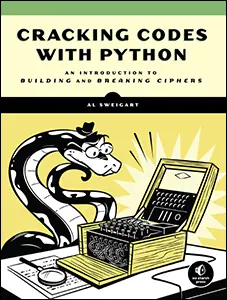# The Invent with Python Blog

Writings from the author of Automate the Boring Stuff.

Sun 20 March 2022

# How Many Recursive Cases and Base Cases Does a Recursive Function Need?

Posted by Al Sweigart in pythonAll recursive functions need at least one recursive case and at least one base case. A recursive case is the set of circumstances where a recursive function calls itself. A base case is the set of circumstances where a recursive function returns without calling itself. Here's a simple recursive factorial function with one base case and one recursive case:

```def factorial(n):
if n == 1:
# BASE CASE
return 1
else:
# RECURSIVE CASE
return n * factorial(n - 1)
```

When you call `factorial(5)`, it returns

If a recursive function has zero recursive cases, it never calls itself and is not a recursive function. The following buggy factorial function has a base case but no recursive case:

```def factorialNoRecursiveCase(n):
if True:
# BASE CASE
return 1
```

When `factorialNoRecursiveCase(5)` is called, it returns `1`. The trouble is, it returns `1` for every argument.

If a recursive function has zero base ases, it never returns and only recurses. This will eventually cause a stack overflow (unless some other . The following buggy factorial function has a recursive case but no base case:

```def factorialNoBaseCase(n):
if True:
# RECURSIVE CASE
return n * factorialNoBaseCase(n - 1)
```

When `factorialNoBaseCase(5)` is called, it causes a `RecursionError: maximum recursion depth exceeded` error.

There is one more requirement for a correctly working recursive function: the recursive function calls must eventually get closer to a base case. Otherwise, the base case is never reached and it will be as though no base case exists, causing a stack overflow. The following buggy factorial function has a recursive case and a base case but never reaches the base case:

```def factorialNoReachBaseCase(n):
if n == 1:
# BASE CASE
return 1
else:
# RECURSIVE CASE
return n * factorialNoReachBaseCase(n + 1)  # Adds one instead of subtracts one!
```

When `factorialNoReachBaseCase(5)` is called, it never reaches the base case and causes a `RecursionError: maximum recursion depth exceeded` error.

When writing your own recursive functions, you should ask yourself three questions:

1. What is the base case?
2. What arguments are passed to the recursive function call?
3. Does the argument get closer to the base case?

If you'd like to learn more about recursion, check out my book, The Recursive Book of Recursion" from No Starch Press.